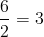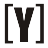# Math Symbols

Below are list of Math Symbols, name, meaning or definition and example of the symbols.

Basic Math Symbol | Geometry | Algebra | Probability & Statistics | Set theory | Logic | Calculus & Analysis | Number | Greek | Roman Numerals

## Basic math symbols

Symbol Symbol Name Meaning / definition Example
+ plus sign addition 1 + 1 = 2
minus sign subtraction 2 − 1 = 1
* asterisk multiplication 2 * 3 = 6
× times sign multiplication 2 × 3 = 6
multiplication dot multiplication 2 ⋅ 3 = 6
÷ division sign / obelus division 6 ÷ 2 = 3
/ division slash division 6 / 2 = 3
horizontal line division / fraction= equals sign/td> equality 5 = 2+3
5 is equal to 2+3
5 is not equal to 4
approximately equal approximation sin(0.01) ≈ 0.01,
xy means x is approximately equal to y
> strict inequality greater than 5 > 4
5 is greater than 4
< strict inequality less than 4 < 5
4 is less than 5
inequality greater than or equal to 5 ≥ 4,
xy means x is greater than or equal to y
inequality less than or equal to 4 ≤ 5,
x ≤ y means x is less than or equal to y
( ) parentheses calculate expression inside first 2 × (3+5) = 16
[ ] brackets calculate expression inside first [(1+2)×(1+5)] = 18
± plus - minus both plus and minus operations 3 ± 5 = 8 or -2
± minus - plus both minus and plus operations 3 ∓ 5 = -2 or 8
mod modulo remainder calculation 7 mod 2 = 1
. period decimal point, decimal separator 2.56 = 2+56/100
ab power exponent 23 = 8
a^b caret exponent 2 ^ 3 = 8
a square root

aa  = a

9 = ±3
3a cube root 3a 3a  ⋅ 3a  = a 38 = 2
4a fourth root 4a 4a  ⋅ 4a  ⋅ 4a  = a 416 = ±2
na n-th root (radical)   for n=3, n8 = 2
% percent 1% = 1/100 10% × 30 = 3
per-mille 1‰ = 1/1000 = 0.1% 10‰ × 30 = 0.3
ppm per-million 1ppm = 1/1000000 10ppm × 30 = 0.0003
ppb per-billion 1ppb = 1/1000000000 10ppb × 30 = 3×10-7
ppt per-trillion 1ppt = 10-12 10ppt × 30 = 3×10-10YoosFuhl.com Would you like to receive notifications on latest updates? No Yes